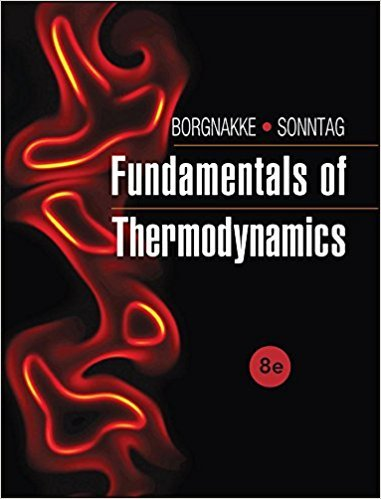×
Get Full Access to Fundamentals Of Thermodynamcs - 8 Edition - Chapter 2 - Problem 53hp
Get Full Access to Fundamentals Of Thermodynamcs - 8 Edition - Chapter 2 - Problem 53hp

×

# Saturated vapor R-410a at 60°C changes volume at constantISBN: 9781118131992 58

## Solution for problem 53HP Chapter 2

Fundamentals of Thermodynamcs | 8th Edition

• Textbook Solutions
• 2901 Step-by-step solutions solved by professors and subject experts
• Get 24/7 help from StudySoup virtual teaching assistantsFundamentals of Thermodynamcs | 8th Edition

4 5 1 304 Reviews
25
0
Problem 53HP

Problem 53HP

Saturated vapor R-410a at 60°C changes volume at constant temperature. Find the new pressure, and quality if saturated, if the volume doubles. Repeat the problem for the case where the volume is reduced to half of the original volume.

Step-by-Step Solution:

Solution 53 HP

Step 1 of 2

We have to the pressure and quality of Saturated vapor R-410a at 60°C when the volume changes to double.

The specific volume of saturated vapour of R-410a at 60o C can be found from the Table.5.1

in the book.0.00497 m3/kgkPa (saturated)

The specific volume of saturated vapour of R-140a, if the volume doubles at constant temperature isSince the mass is constant, specific volume also doubles when the volume doubles.= 0.00994 m3/kg superheated vapour

The pressure of the R-140 at 60oC  and with specific volume 0.00994 m3/kg can be found by interpolating between 2000 kPa and 3000 kPa from the Table B.5.2 in the book.m3/kg at 2000 kPa andm3/kg at 3000 kPa= 2000+(1000)(0.8)

= 2800 kPa

The quality is 1 as it is in vapour state only.

Therefore, the new pressure and quality are 2800 kPa and 1.

Step 2 of 2

##### ISBN: 9781118131992

Unlock Textbook Solution